# Inductor for Power LinesIntroduction of the LQM series - ideal power inductors for Bluetooth® Low Energy power supplies

## 1The meaning of Bluetooth® Low Energy

Up until now, Bluetooth® has been popular as a means of short-distance audio communication, such as wireless headphones, etc.

Bluetooth® Low Energy, which is used in such applications as IoT with power savings over Bluetooth. Bluetooth® Low Energy is a specification added in Bluetooth® 4.0.

Like conventional Bluetooth®, communication is performed using radio waves of the 2.4 GHz band; however, in comparison to conventional Bluetooth®, low power consumption is achieved by suppressing the transfer speed and the communication range.

## 2Recommended power inductors for Bluetooth® Low Energy

Many devices for IoT applications are small in size, such as beacons or active trackers, and this creates demand for power inductors that are compact and cost-effective.

Here we present power inductor products that we recommend for use in Bluetooth® Low Energy applications. The 0603-inch size LQM18DN100M70 and LQM18PNR47NFR employ a ferrite core with a multilayer structure.
In particular, the LQM18DN100M70 is a new product that leverages Murata’s multilayer technology, by providing low Rdc while delivering high inductance of 10 µH and superior DC superimposition characteristics.

Table 1: Power inductors recommended for use with Bluetooth® Low Energy
Switching frequency of built-in DC-to-DC converter Size code Product number Inductance value
1MHz 0603-inch size LQM18DN100M70 10µH
4MHz LQM18PNR47NFR 0.47µH

Later in our descriptions, we will explain the reason for recommending these parts for use with Bluetooth® Low Energy.

## 3Required characteristics for power inductors used in Bluetooth® Low Energy

The required voltage for Bluetooth® Low Energy is made available by the DC-DC converter built into the Bluetooth® Low Energy IC.

Under these circumstances, the power inductor for voltage conversion will be connected externally, but depending on the performance of the power inductor used here the power conversion efficiency of the DC-DC converter will vary.
Since the emphasis is on power efficiency in Bluetooth® Low Energy applications, it is important to connect an external power inductor with superior power conversion efficiency.

Here, consideration is given to the necessary performance of power inductors suited to Bluetooth® Low Energy, and actual products are introduced.

## 4An investigation of the effects on power conversion efficiency of differing inductor characteristics

We investigated how differences among inductors affect the power conversion efficiency of Bluetooth® Low Energy applications.
Here, attention is paid to the DC losses and the AC losses that exist in inductors. DC losses are losses that are attributable to the DC component of the current flowing in the inductor, and are expressed below using the DC resistance (Rdc) and the DC component of the current (Idc).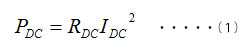On the other hand, AC losses are losses that are attributable to mainly the AC component of the current flowing in the inductor, and are expressed below using the AC resistance (Rac), which is the apparent resistance due to the winding resistance plus the core losses, and the AC component of the current (Irms).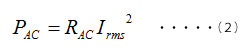The AC component (Irms) expresses the size of the current amplitude, and this value can be reduced by increasing the switching frequency or increasing the inductance of the power inductor.

As of 2020 the switching frequencies of most DC-DC converters incorporated into Bluetooth® Low Energy ICs were either around 1 MHz or 4 MHz. We ran a simulation to determine the ratio of DC loss and AC loss when using inductors with rated inductances of 1.5 µH and 10 µH at each frequency (Figure 1).

Although the frequency conditions and other factors also have an influence, Rac increases more than Rdc in the power inductor. For this reason, in the small area in which the load current (equal to Idc) is 50 mA or less as shown in Figure 1, we see that the DC loss becomes very small and the AC loss occupies a large portion.

From these results, in order to reduce the inductor loss in Bluetooth® Low Energy applications, the inductance, which affects AC loss, and the Rac become important parameters.
At low Rac it is ideal to obtain high inductance; however, in trying to obtain inductance the winding resistance and core losses will be increased. For this reason, the balance between inductance and Rac becomes important.
In regard to Rdc, in the Bluetooth® Low Energy environment where there is a small proportion of DC loss, the degree of influence may be considered to be low. However, when Rdc becomes overly large it cannot be ignored and attention must be paid to this.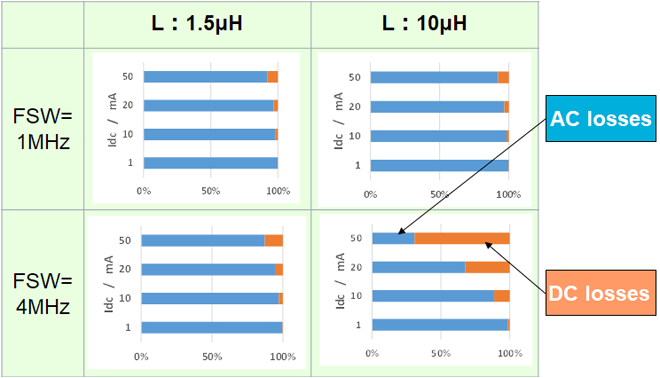Figure 1: Proportion of AC loss to DC loss of a power supply IC for Bluetooth® Low Energy

As causes of DC-DC converter power losses, in addition to inductor losses, other losses that can be considered include switching losses of the IC, and IC continuity losses.
Concerning power inductors intended for Bluetooth® Low Energy, since the size of the inductance may influence the switching loss, this point must also be considered in the selection.

Figure 2 is a simulation of the current waveforms that flow in the inductor.
To boost power conversion efficiency when operating under the light loads that characterize Bluetooth® Low Energy applications, a control mode called pulse frequency modulation (PFM) control is typically used. PFM control increases power conversion efficiency by reducing the number of times switching occurs by not performing switching continuously. The left chart of Figure 2 shows the current waveform when the inductance has been changed at the same frequency.

Larger inductance will keep down the size of the current amplitude; however, the number of triangular waves generated will increase to adjust the total current amount. This signifies an increase in the number of switching cycles, and there will be an increase of the switching loss. Similarly, the right chart of Figure 2 shows the current waveform when the frequency has been changed with the same inductance. Higher frequencies will keep down the size of the current amplitude; however, it is understood that the number of switching cycles will increase.

In this way, in PFM control, by increasing the inductance or the frequency and making the current amplitude smaller, on one hand the AC losses can be reduced, but there is also the aspect of increasing the switching losses.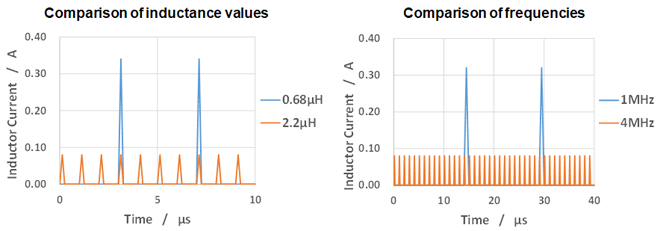Figure 2: Current waveform when the inductance value or the switching frequency is changed

Well then, how much inductance would be good to use?
Figure 3 shows the results of a simulation of the DC-DC converter loss when the inductance value is changed, at switching frequencies of 1 MHz and 4 MHz. Using the characteristics of the LQM21P-GH series as the basis, we calculated the change in Rac as well when the inductance value changes.
The graphs show the total loss of the DC-DC converter and the loss for the inductor alone.

In the case of the low 1 MHz switching frequency, it can be seen that as the inductance became larger the inductor loss dropped, and accompanying this the total losses also dropped.
On the other hand, in the case of 4 MHz, as the inductance became larger the inductor loss dropped, but the result was that the total losses increased. The reason for this is that as the inductance became larger, the switching losses increased as a result.
In the case of 4 MHz compared to 1 MHz, because the number of switching cycles is large, the proportion of switching loss is large, and so it can be said that the increase of switching loss appeared remarkable due to the change of the inductance.

Based on these results, we determined that the appropriate inductance value for reducing total loss is around 10 µH at 1 MHz and 0.47 µH to 1.0 µH at 4 MHz.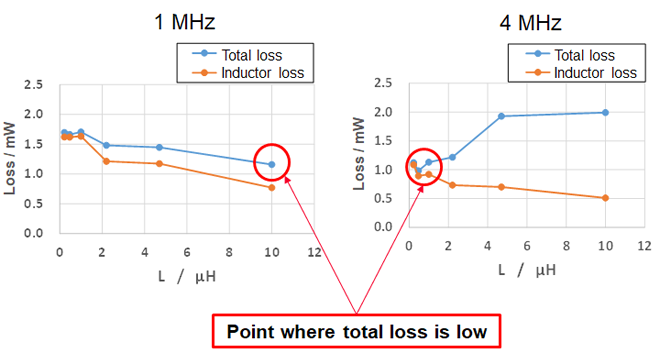Figure 3: Inductance value and power loss

## 5Summary regarding inductors suited for Bluetooth® Low Energy

Figure 4 summarizes the inductor products suitable for Bluetooth® Low Energy applications.
As explained above, it can be said that inductance on the order of 10 µH is suitable for a switching frequency of 1 MHz, and inductance from 0.47 µH to 1.0 µH is suitable for 4 MHz.

[1 MHz]

Low Rac at inductances of 10 µH and higher is important.
Recommended inductor：LQM18DN100M70

[4 MHz]

Low Rac at inductances of 0.47 µH to 1.0 µH is important.
Recommended inductor：LQM18PNR47NFR

Figure 4: Summary of necessary power inductor characteristics

## 6Performance of inductors selected for use with Bluetooth® Low Energy

We used an evaluation board for Bluetooth® Low Energy applications to do a comparative evaluation of power consumption with different power inductors. The results were different even for inductors with the same rated inductance due to differences in characteristics such as DC resistance and magnetic properties.

Figure 5 shows results when using a switching frequency of 1 MHz. The LQM18DN100M70 is a new product that leverages Murata’s electrode forming technology and multilayer technology to achieve both low Rdc and superior DC superimposition characteristics. As you can see, it dramatically reduces power consumption compared with the earlier LQM18FN100M00. It also delivers characteristics superior to those of products from other manufacturers.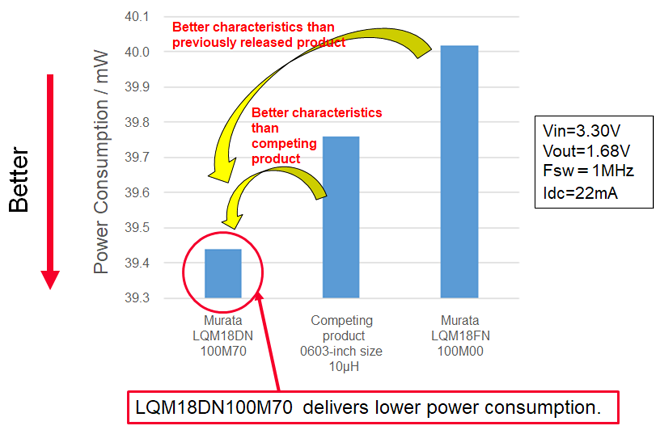Figure 5: Comparison of power consumption at switching frequency of 1 MHz

Figure 6 shows results when operating at a switching frequency of 4 MHz. As you can see, under these operating conditions an inductance value lower than 10 µH results in reduced inductor loss. In the Murata product lineup the LQM18PNR47NFR will deliver the lowest power consumption.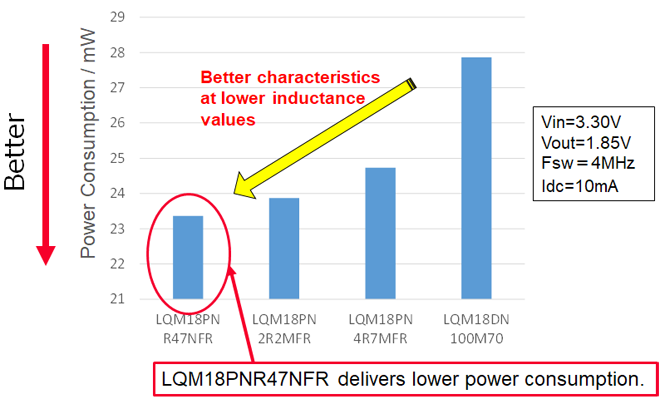Figure 6: Comparison of power consumption at switching frequency of 4 MHz

## 7Summary

Since power inductors used in Bluetooth® Low Energy applications affect power conversion efficiency, they are a key component influencing low power consumption, a key feature of Bluetooth® Low Energy technology.

The specifications sought in an inductor will differ depending on the switching frequency of the power IC used in Bluetooth® Low Energy. At 1 MHz, the value will be on the order of 10 µH, and at 4 MHz, on the order of 0.47 µH to 1.0 µH.

However, even among products with the same configuration and inductance value, the power conversion efficiency differs depending on the inductor series. Therefore, it is necessary to select the inductor series that is best suited for Bluetooth® Low Energy applications.

### Here are the optimum power inductors for Bluetooth® Low Energy that were introduced

Supporting a 1 MHz switching frequency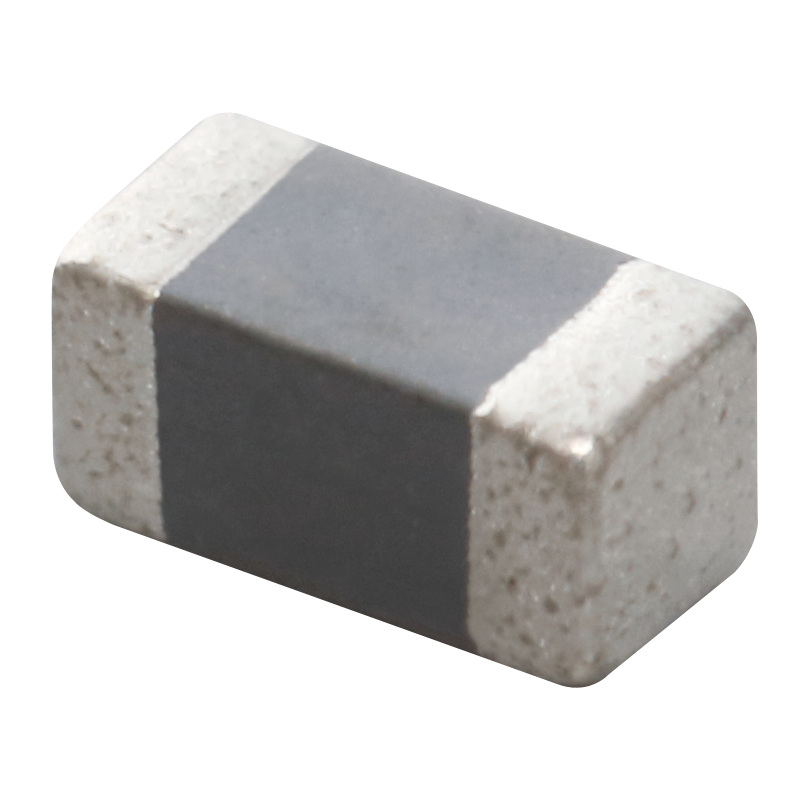Supporting a 4 MHz switching frequency